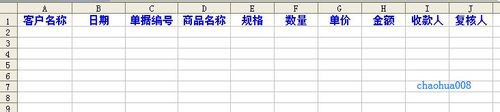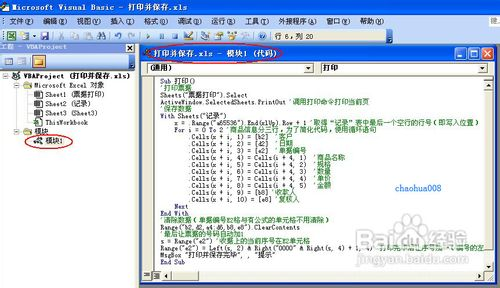时间：2020-02-10  来源：百度经验  作者：chaohua008  阅读：4

C7单元格是数量汇总公式=SUM(C4:C6)

E4单元格是计算数量乘单价的金额公式=C4*D4，把这个公式向下拖动复制到E5与E6单元格。

E7单元格是金额汇总公式=SUM(E4:E6)Sub 打印()

'打印票据

Sheets("票据打印").Select

ActiveWindow.SelectedSheets.PrintOut '调用打印命令打印当前页

'保存数据

With Sheets("记录")

x = .Range("a65536").End(xlUp).Row + 1 '取得“记录”表中最后一个空行的行号（即写入位置）

For i = 0 To 2 '商品信息分三行，为了简化代码，使用循环语句

.Cells(x + i, 1) = [b2] '客户

.Cells(x + i, 2) = [d2] '日期

.Cells(x + i, 3) = [e2] '单据编号

.Cells(x + i, 4) = Cells(i + 4, 1) '商品名称

.Cells(x + i, 5) = Cells(i + 4, 2) '规格

.Cells(x + i, 6) = Cells(i + 4, 3) '数量

.Cells(x + i, 7) = Cells(i + 4, 4) '单价

.Cells(x + i, 8) = Cells(i + 4, 5) '金额

.Cells(x + i, 9) = [b8] '收款人

.Cells(x + i, 10) = [e8] '复核人

Next

End With

'清除数据（单据编号E2格与有公式的单元格不用清除）

Range("b2,d2,a4:d6,b8,e8").ClearContents

'最后让票据的号码自动加1

s = Range("e2") '收据上的当前序号在E2单元格

Range("e2") = Left(s, 2) & Right("0000" & Right(s, 4) + 1, 4) '打印完毕后让序号加一（编号的左两位是字符，右四位是数字）

MsgBox "打印并保存完毕", , "提示"

End Sub